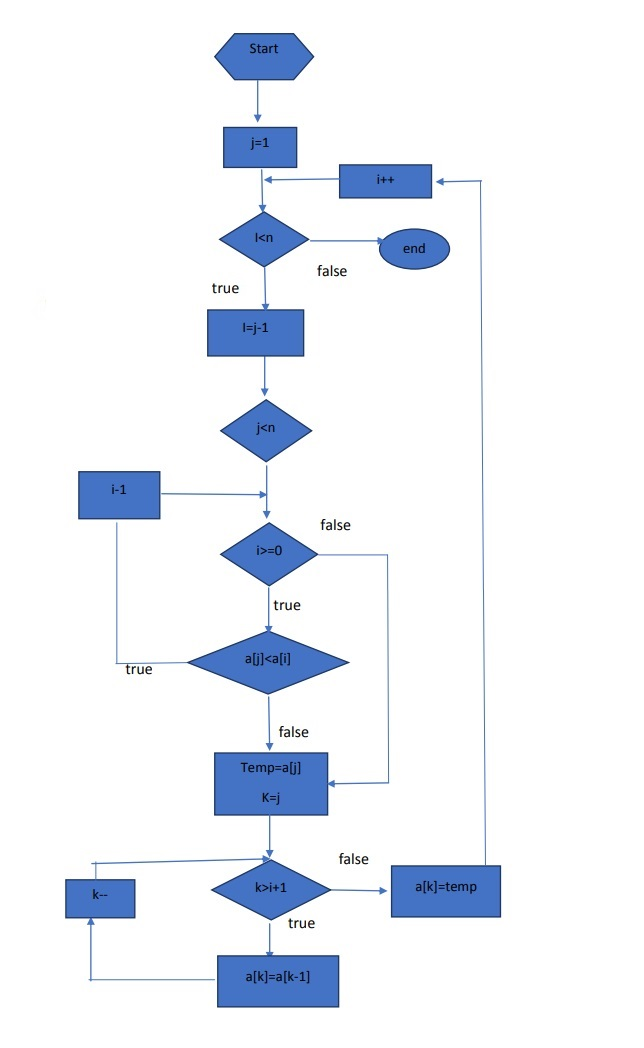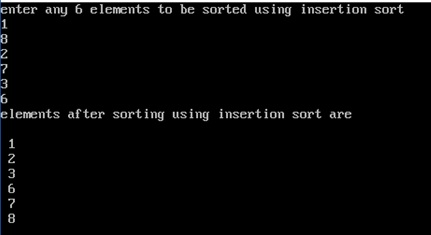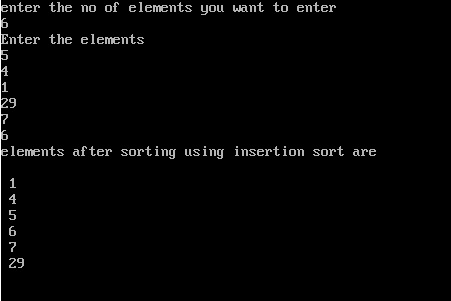# Insertion sort Algorithm, flowchart and C, C++ Code

In this article, we are going to learn about Insertion Sort, its algorithm, flow chart and C, C++ program to implement Insertion sort.
Submitted by Raunak Goswami, on August 12, 2018

In the last article, we discussed about the bubble sort with algorithm, flowchart and code. In this article, we are going to discuss about another basic sorting technique i.e. insertion sort.

As the name suggests the sorting is done by using successive insertions of the key element selected by the sorting algorithm at its correct place. As the sorting begins the key element chosen is always the second element so that there can be at least one element at the left of the key element to be compared. After comparison the key element is swapped with the element at its left if required and the key element is changed to the element at the immediate right of the previous key element after that the key element is again compared with the elements at it left and the element is swapped with the key element after comparison if required, if the sorting is done in ascending order then the key element should always be greater than the element at its left if not, then the swapping is performed with key element and vice versa for the descending order.

Let us look at the algorithm of insertion sort for a better understanding of the logic to be used:

```    Insertion sort(a[],n)
for j->2 to n
do key <-a[j]
i<-j-1
while i>=0 and a[i]>key
do a[i+1]<-a[i]
i<-i+1
a[i+1]<-key
end
```

The algorithm can also be explained in the form of a flowchart as follows:### C code for Insertion sort

```#include<stdio.h>

int main()
{
int a;
int key;
int i,j;
int temp;

printf("Enter any six elements to be sorted using insertion sort\n");
for(i=0;i<6;i++)
{
scanf("%d",&a[i]);
}

for(j=1;j<6;j++)
{
key=a[j];
i=j-1;
while((i>=0)&&(a[i]>=key))
{
temp=a[i+1];
a[i+1]=a[i];
a[i]=temp;
i=i-1;
}
a[i+1]=key;
}

printf("elements after sorting using insertion sort are \n");
for(i=0;i<6;i++)
{
printf("%d  \n",a[i]);
}

return 0;
}
```

Output### C++ code for Insertion sort

```#include <iostream.h>
using namespace std;

int main()
{
int key,i,j,temp;
int c,a;

cout<<"enter the no of elements you want to enter"<<endl;
cout<<c<<endl;

cout<<"Enter the elements"<<endl;
for(i=0;i<c;i++)
{
cin>>a[i];
}

for(j=1;j<c;j++)
{
key=a[j];
i=j-1;
while((i>=0)&&(a[i]>=key))
{
temp=a[i+1];
a[i+1]=a[i];
a[i]=temp;
i=i-1;
}
a[i+1]=key;
}

cout<<"elements after sorting using insertion sort are \n"<<endl;
for(i=0;i<6;i++)
{
cout<<" "<<a[i]<<endl;
}

return 0;
}
```

OutputWhat's New

Top Interview Coding Problems/Challenges!

Languages: » C » C++ » C++ STL » Java » Data Structure » C#.Net » Android » Kotlin » SQL
Web Technologies: » PHP » Python » JavaScript » CSS » Ajax » Node.js » Web programming/HTML
Solved programs: » C » C++ » DS » Java » C#
Aptitude que. & ans.: » C » C++ » Java » DBMS
Interview que. & ans.: » C » Embedded C » Java » SEO » HR
CS Subjects: » CS Basics » O.S. » Networks » DBMS » Embedded Systems » Cloud Computing
» Machine learning » CS Organizations » Linux » DOS
More: » Articles » Puzzles » News/Updates